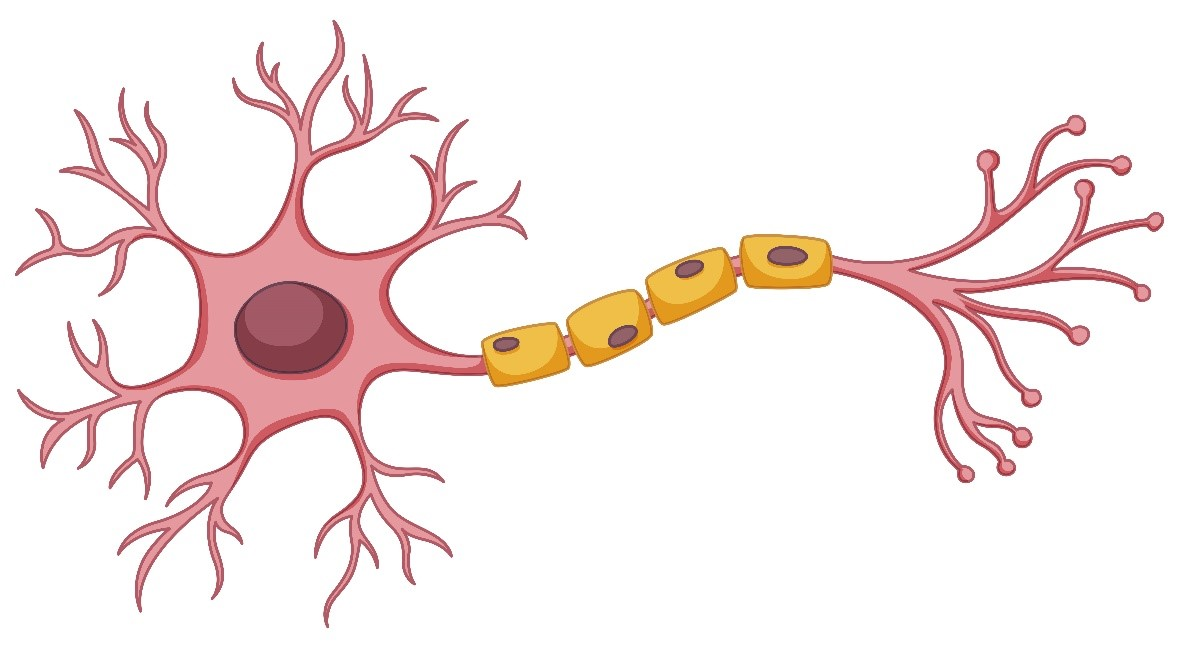• by Team Handson
• September 20, 2022

## Artificial Neural Networks: What they are and how it works

»In this writing we will explain what a neural network is, how it works and the concept behind it.

»Artificial neural networks are based on the functioning of networks of biological neurons. The neurons that we all have in our brain are made up of dendrites, the soma and the axon: The dendrites are responsible for capturing the nerve impulses that other neurons emit. These impulses are processed in the soma and transmitted through the axon that emits a nerve impulse to the neighbouring neurons.What is a neural network in Artificial Intelligence?

At a schematic level, an artificial neuron is represented as follows:

»In the circumstance of artificial neurons, the summation of the inputs multiplied by their allied weights governs the “nerve impulse” that the neuron collects. This value is processed inside the cell by means of an activation function that returns a value that is sent as an output of the neuron. In the same way that our brain is made up of neurons interconnected with each other, an artificial neural network is made up of artificial neurons connected with each other and gathered at diverse levels that we call layers.

» A layer is a set of neurons whose inputs come from a previous layer (or from the input data in the case of the first layer) and whose outputs are the input of a later layer.

In the following image we can see a network with four layers:The neurons of the first layer receive as input the real data that feeds the neural network. That is why the first layer is known as the input layer. The last layer is visible result of the network, so the last layer is known as the output layer. The layers between the input layer and the output layer are known as hidden layers since we do not know both the input and output values.

A neural network, therefore, is always composed of an input layer, an output layer (if there is only one layer in the neural network, the input layer matches the output layer), and can comprise of 0 or more layers hidden. The concept of Deep Learning is born as a result of using a large number of hidden layers in networks.

How is a neural network trained?

Working on a neural network involves correcting each of the weights of the inputs of all the neurons that are fragment of the neural network, so that the answers of the output layer adjust as closely as probable to the data we know.

We can see a very simplified example of the process of training a network for the detection the thickness of each arrow represents the weight that input has in the neural network. As we can understand, as the weights are accustomed, the error reduces.

If we want the neural network to be able to generalize and identify dogs in any image, it is important to use a large number of images for training, both images that are dogs (labelled as 1) and images that are not dogs (labelled 0), including as much variability as possible. With this, the network will be able to adjust its parameters to satisfy all the images as much as possible, so it will be able to accurately extract the characteristics that identify the presence of a dog in an image.